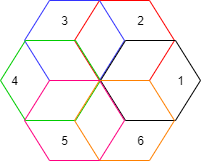# PWM Gate Signal Generator (Three-phase, Three-level)

Generate twelve switch-controlling pulses for three-phase, three-level gating switching devices

• Library:
• Simscape / Electrical / Control / Pulse Width Modulation

•## Description

The PWM Gate Signal Generator (Three-phase, Three-level) block controls switching behavior for a three-phase, three-level power converter. The block uses the gating times provided as inputs to generate twelve switch-controlling pulses. You can obtain the gating time information using the PWM Timing and Waveform Generator (Three-phase, Three-level) block.

### Simplified Space-Vector PWM Method

To determine the switching sequence and the duration time of the voltage vector, the PWM Gate Signal Generator (Three-phase, Three-level) block uses a simplified space-vector pulse width modulation (SVPWM) method on the space-vector diagram of a three-level inverter .

This figure shows the six small hexagons that comprise the space-vector diagram of a three-level inverter:Each of these six small hexagons represents the space-vector diagrams of a two-level inverter, with the diagrams centered on the six vertices of an inner small hexagon, as shown in this figure:To simplify into the space-vector diagram of a two-level inverter, you must select one of the small hexagons by specifying a value at the SmallHexNum port. The block then transforms the three-level space-vector plane into a two-level space-vector plane by subtracting the original reference voltage vector by the amount of the center voltage vector of the hexagon you choose.

### Sampling Mode

This block allows you to choose natural, symmetric, or asymmetric sampling of the modulation wave.

The PWM Gate Signal Generator (Three-phase, Three-level) block does not perform carrier-based pulse width modulation. Instead, the block uses the gating times to generate both the switch-controlling pulses and the modulation waveforms that the block outputs.

Carrier-based PWM is, however, useful for showing how the sampling mode that you select relates to the switch-on and switch-off behavior of the pulses that the block generates. A generator that uses a three-level, carrier-based PWM method:

1. Samples a reference wave

2. Compares the sample to two parallel triangle carrier waves, separated by one level3. Generates a switch-on pulse if a sample is higher than the carrier signal or a switch-off pulse if a sample is lower than the carrier wave

To determine switch-on and switch-off pulse behavior, a three-level carrier-based PWM generator uses these methods to sample each of the triangle waves:

• Natural — The sampling and comparison occur at the intersection points of the modulation wave and the carrier wave.• Asymmetric — Sampling occurs at the upper and lower boundaries of the carrier wave. The comparison occurs at the intersection that follows the sampling.• Symmetric — Sampling occurs only at the upper boundary of the carrier wave. The comparison occurs at the intersection that follows the sampling.## Ports

### Input

expand all

Switch-on gating time, specified as a vector of three elements, with one element for each phase.

Switch-off gating time, specified as a vector of three elements, with one element for each phase.

Number of one of the small hexagons that composes the space–vector diagrams of conventional two-level inverters, specified as a scalar between 1 and 6. For more information, see the Simplified Space-Vector PWM Method section.

### Output

expand all

Twelve pulse waveforms that determine switching behavior in the attached power converter, returned as a vector.

## Parameters

expand all

Wave-sampling method. The sampling mode determines whether the block samples the modulation waveform when the waves intersect or when the carrier wave is at one or both of its boundary conditions.

Rate at which the switches in the power converter switch.

Time interval between successive block executions. To ensure adequate resolution in the generated signal, set this value to be less than or equal to `1/(50*Fsw)`, where Fsw is the Switching frequency (Hz).

 Chung, D. W., J. S. Kim, and S. K. Sul. “Unified Voltage Modulation Technique for Real Time Three-Phase Power Conversion.” IEEE Transactions on Industry Applications, Vol. 34, No. 2, 1998, pp. 374–380.

 Seo, J. H., C. H. Choi, and D. S. Hyun. “A new simplified space-vector PWM method for three-level inverters.” IEEE Transactions on Power Electronics, Vol. 16, No. 4, 2001, pp. 545-550.